# 16 Presentation of Work by T. Taniuchi

Ryoyu Utiyama

#### DOI

10.34663/9783945561294-23

#### Citation

Utiyama, Ryoyu (2011). Presentation of Work by T. Taniuchi. In: The Role of Gravitation in Physics: Report from the 1957 Chapel Hill Conference. Berlin: Max-Planck-Gesellschaft zur Förderung der Wissenschaften.

UTIYAMA: I am going to talk about an article of my colleague, Dr. T. Taniuchi.

In quantizing the gravitational field, or any type of non-linear fields, particular attention should be paid to the following points.

In general a disturbance of non-linear fields in Minkowski-space propagates with a super-light velocity in some cases and with a sub-light velocity in some other cases. Namely, the velocity of non-linear fields usually depends on the strength of fields. This means that it is not so clear as in the case of linear fields whether any couple of field-quantities on a space-like surface is commutable or not. Another remarkable feature of non-linear fields is the following. Suppose that some initial value is given which has no discontinuity on the initial spacelike surface. In some cases, however, some discontinuity of the field strength (shock wave) may be expected to occur after the time passed. On the contrary, even though the initial value has some discontinuity, at a later time such a discontinuity will be expected to be smeared out owing to the non-linearity. In general the occurrence of shock waves depends not only on the type of non-linear fields but also on the type of initial values.

Owing to these situations mentioned above, it seems necessary to investigate the character of propagation of the gravitational field before making quantization of this field.

However, since the gravitational field is too complicated to deal with, we considered the following two cases as our training, the Born-type field with the Lagrangian density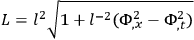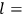constant with a dimension (length)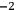,

and the Landau-Khalatnikow-type field [2,3]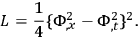These two types of non-linear field were adopted by Heisenberg and Landau respectively in order to explain the phenomena of multiple meson-productions.

Our method of solving these equations is completely similar to that of Courant and Friedrichs stated in their textbook . We are also planning to investigate the equation of gravitational field in some simple case.

(1) Case of Born-type field:: The Lagrangian density is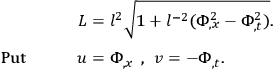Then the field equation is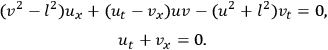In this case the two kinds of characteristics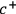-curves and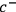-curves consist of two sets of parallel straight lines respectively and shock waves do not occur.

Fig. 16.1: Solution of Born-type field

Consider the following initial condition: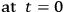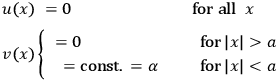The solution in this case is shown in Figure 16.1, where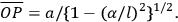The initial discontinuity at A propagates with the light velocity. The feature of propagation is quite similar to that of linear fields, because this particular non-linear field becomesidentical with the linear field in case of an extremely weak field.

(2) Case of Landau-Khalatnikow-type field:: The Lagrangian density is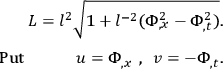Fig. 16.2: Initial value for L-K-type field

Let us consider the two kinds of initial values shown in Figure 16.2. In the case of (a), namely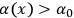, the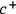-characteristics intersect with each other and the shock wave occurs in spite of the continuous initial value (see Figure 16.3). On the other hand, in the case of (b), namely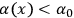, the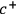-characteristics diverge from each other, that is, the rarefaction wave occurs (see Figure 16.4).

Let us also consider the same initial condition as that of Born-type field. The solution is illustrated in Figure 16.5. In this case the discontinuity of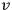at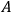is smeared out, and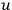and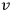at any later time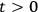continuously vanish on the light-cone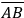. Contrary to linear fields, the disturbance of the field soaks into the region CPC'.

From these results we can see that the latter type of field is more adequate to the explanation of the phenomena of multiple meson-production.

Fig. 16.3: Characteristics for (a)